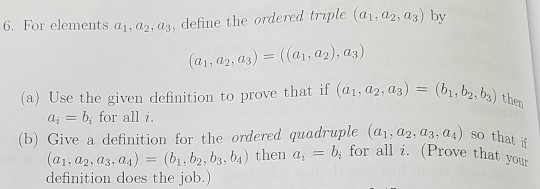# Question 6. For elements ai, аг, аз, define the ordered triple (ai, a2,as) by (al, di 2 , as)-((al, a2),a3) (a) Use the given definition to prove that if (41, a2,as)-(bi, b2 b ) t 2, b3) t en ai = bi for all i. (b) Give a definition for the ordered quadruple (ai,a2, a3, a4) 8o (Prove that,at f (ai, a2, аз . a1)-(bi, b2, b, b1) then ai-bi for all i. definition does 6. For elements ai, аг, аз, define the ordered triple (ai, a2,as) by (al, di 2 , as)-((al, a2),a3) (a) Use the given definition to prove that if (41, a2,as)-(bi, b2 b ) t 2, b3) t en ai = bi for all i. (b) Give a definition for the ordered quadruple (ai,a2, a3, a4) 8o (Prove that,at f (ai, a2, аз . a1)-(bi, b2, b, b1) then ai-bi for all i. definition does the job. yoHNYJCB The Asker · Other MathematicsHelp for this problem would be appreciated thanks

Transcribed Image Text: 6. For elements ai, аг, аз, define the ordered triple (ai, a2,as) by (al, di 2 , as)-((al, a2),a3) (a) Use the given definition to prove that if (41, a2,as)-(bi, b2 b ) t 2, b3) t en ai = bi for all i. (b) Give a definition for the ordered quadruple (ai,a2, a3, a4) 8o (Prove that,at f (ai, a2, аз . a1)-(bi, b2, b, b1) then ai-bi for all i. definition does the job. yo
More
Transcribed Image Text: 6. For elements ai, аг, аз, define the ordered triple (ai, a2,as) by (al, di 2 , as)-((al, a2),a3) (a) Use the given definition to prove that if (41, a2,as)-(bi, b2 b ) t 2, b3) t en ai = bi for all i. (b) Give a definition for the ordered quadruple (ai,a2, a3, a4) 8o (Prove that,at f (ai, a2, аз . a1)-(bi, b2, b, b1) then ai-bi for all i. definition does the job. yo